Mobile QR Code1. (School of Electrical Engineering and Computer Science, Gwangju Institute of Science and Technology)

Multi-phonon emission process, first principles calculation, density functional theory

## I. INTRODUCTION

Point defects cause unintentional physical effects on semiconductor devices. Especially, in the MOS (metal-oxide-semiconductor) structure the bulk and interface defects degrade device performance and stability[2,3]. These defects act as charged traps or recombination centers. For the silicon-based electronic devices, the non-radiative recombination is the major mechanism of the defect-induced trapping process. Despite of practical importance and tremendous research efforts, rigorous analysis of defects in the semiconductor devices is still a difficult task.

The non-radiative multiphonon emission (MPE) has been widely adopted to describe the non-radiative trapping process. Many studies have made theoretical contributions over the last 60 years[6-8]. A common problem of these models is that the calculated result sensitively depends on model formulations. This problem is solved by evaluating electron-phonon coupling constants via the first-principles calculation. By first principles calculation based approaches, physical quantities like the formation energy and the transition level of a charged defects can be obtained.

These methods have been successfully applied to obtain non-radiative recombination coefficients of III-V semiconductor systems[12-. In this paper, we apply the calculation method to the Si bulk and the Si-SiO2 interface. In this study, the Si bulk model is used to check the physical soundness of our first principle based MPE calculation method. The Si-SiO2 interface structure model is considered to analyze the non-radiative recombination phenomena when defects are formed at the oxide-semiconductor interface.

This paper is organized as follows. In Sec II, the theoretical formulation based on the first principle approach is explained in detail. The calculation workflows and computational tools for the calculation are also introduced. In Sec III, the atomistic structures with defects for the calculation are introduced. In Sec IV, results of the MPE calculation are presented. In Sec V, conclusions are drawn.

## II. Theoretical Formulation and Calculation Method

First principles-based calculations are powerful tools for material properties calculations . The density functional theory (DFT) calculation based on the Kohn-Sham equation is used as the most popular first principles calculation method with the accuracy of calculation and the reasonable computational cost . In this paper, we have calculated the characteristics of defect structures required for MPE calculation through the DFT approach.

The calculation is performed for the atomistic supercell structures with defects. All calculations are derived from the results of a pristine structure without a defect and a charged or neutral structure with defects. The thermodynamic formation energy of a defect and its transition levels are key factors to the MPE simulation workflow.

The formation energy $E^{f}\left[X^{q}\right]$ of a defect $X$ at charge state $q$ in a charge state $q$ is defined as 

##### (1)
$E^{f}\left[X^{q}\right]=E_{t}\left[X^{q}\right]-E_{t}[b u l k]-\sum_{i} n_{i} \mu_{i}+q E_{F}+E_{c o r r}$

where $E_{t}\left[X^{q}\right]$ is the total energy derived from a supercell containing defect X and $E_{t}[b u l k]$ is the total energy of defect-free crystalline supercell. The integer $n_{i}$ is the number of each defect type and $\mu_{i}$ is the chemical potential. $E_{\text { corr }}$ is a correction term for the charge interaction between neighboring periodic supercells. Fig. 1 schematically shows the formation energy and its transition levels in the bandgap as functions of the Fermi energy level $E_{F}$.

Fig. 1. Schematic illustration of the formation energy $E^{f}$ versus the Fermi level $E_{F}$ for a defect X for three charged states ($q :-1,0,1$)).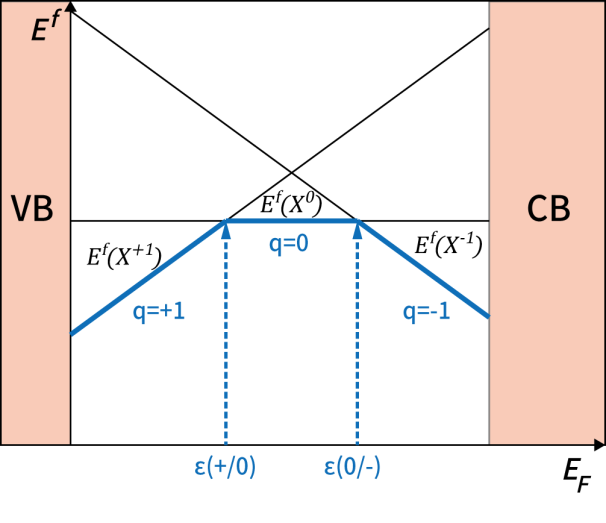The thermodynamic transition level $\varepsilon\left(q_{1} / q_{2}\right)$ is defined as the Fermi-level position for which the formation energies of charge states $q_{1}$ and $q_{2}$ are equal (cross point):

##### (2)
$\varepsilon\left(q_{1} / q_{2}\right)=\frac{E^{f}\left(X^{q_{1}} ; E_{f}=0\right)-E^{f}\left(X^{q_{2}} ; E_{f}=0\right)}{q_{2}-q_{1}}$

The thick blue lines represent the minimum energies that each cell with a defect can have, depending on the charge state of the defect. The intersecting point is the transition level according to the pair of charge states.

Fig. 2 shows a schematic diagram illustrating how transitions are made through the MPE process. Let us consider a MPE process shown in Fig. 2(a). The defect energy with respect to the configuration coordinate is shown in Fig. 2(b). $\Delta Q$ is the distance between the defect and the neighboring (electrically interacting) atom. In Fig. 2(a), $\Delta E$, which means the minimum ionization energy level of the hole, is defined as the closest transition level to the valence band in the supercell calculation of the defect. (Position of $\varepsilon(+/ 0)$ in Fig. 1) In Fig. 2(b), the $\Delta Q$ value shows the distance between adjacent atoms of the defect in the supercell calculation. The curves in Fig. 2(b) are indicate the potential curves in neural (black, $A^{0}$) and negative charged defect ($A^{0+h^{+}}$). The hole carrier capture process is occurred by a vibrational relaxation process between the two potential curves[8,11].

Fig. 2. Illustrations of non-radiative multiphonon emission transition process (a) band diagram, (b) configuration diagram. In this illustration, the defect is a deep acceptor with a negative and a neutral charge state. $\Delta E$ is the ionization energy of the acceptor, and $Q$ is relative defect configuration coordinate.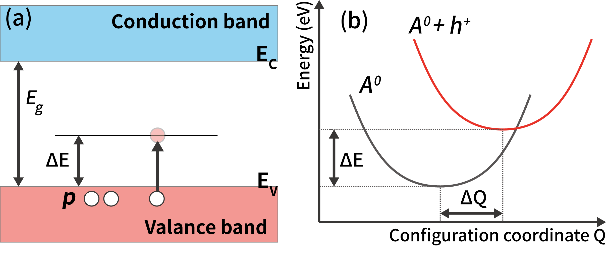Recently, several calculation methods are suggested based on the first principles-based MPE transition scheme[8,17,18]. In this study, we employ a method based on the adiabatic approximation. The electron transition rate between electron states $s$ and $l$ can be expressed as

##### (3)
$W_{s l}=\frac{\left|C_{s l}^{k}\right| \omega_{k}(2 \pi)^{\frac{1}{2}}}{2 \hbar D} \times \sum_{k} \left[ \begin{array}{c}{\left[\operatorname{coth} y_{k}+1\right) \exp \left(-\frac{\left(\Delta E_{s l}-\hbar \omega_{k}-E_{M}\right)^{2}}{2 D^{2} \hbar^{2}}\right)+} \\ {\left(\operatorname{coth} y_{k}-1\right) \exp \left(-\frac{\left(\Delta E_{s l}+\hbar \omega_{k}-E_{M}\right)^{2}}{2 D^{2} \hbar^{2}}\right)}\end{array}\right]$

where $\Delta E_{s l}$is the total energy change between the atomic relaxed $s$ and $l$ electron states. (same as $\Delta E$ in Fig. 2) $k$ is the index of phonon mode with frequency $\omega_{k}$. Also, $D^{2}=\frac{1}{2} \sum_{J} \omega_{j}^{2} \Delta_{j}^{2}\left(2 \overline{n_{j}}+1\right)$, $\overline{n_{j}}= \left[\exp \left(\beta \hbar \omega_{j}\right)-1\right]^{-1}$, $\Delta_{j}=\left(\frac{M_{j} \omega_{j}}{\hbar}\right)^{\frac{1}{2}}\left(Q_{j}^{0(s)}-Q_{j}^{0(l)}\right)$, $y_{k}= \beta \hbar \omega_{k} / 2$, and $\beta=(k T)^{-1}$. The relaxed ground state coordination of phonon mode $j$ are $Q_{j}^{0(s)}$ and $Q_{j}^{0(l)}$ when electron states $s$ and $l$. Electron states s and l are the lowest charged transition level in the bandgap and the first three valence bands, respectively. All electron states are evaluated from the first principle calculation of the structure with the defect. The reorganization energy of relaxed atom after electronic transition is $E_{M}=\frac{1}{2} \sum_{j} \hbar \omega_{j} \Delta_{j}^{2}$. All parameters come from the first principles-based calculation and its analysis.

The electron-phonon coupling constant in (3) between electronic states $s$ and $l$ and phonon mode $k$ is

##### (4)
$C_{s l}^{k}=\frac{i \hbar \sum_{R} \mu_{k}(R) \psi_{s}\left|\frac{\partial H}{\partial R}\right| \psi_{l}}{\Delta E_{s l}}$

where $\mu_{k}(R)$ is the k-th phonon mode, $\psi_{s}$ and $\psi_{l}$ are electronic wave functions of electron states $s$ and $l$, and $H$ is the system Hamiltonian of the supercell with the defect. The capture coefficient is obtained by multiplying the transition rate $W_{s l}$ and volume of the supercell, $V$. ($C_{p}=V W_{s l}$)

In this work, as a preliminary study, only the gamma point in the momentum space and the first three phonon modes are used in the calculation. Most of the transitions in shallow charged traps depend on low energy phonon modes. These phonon dispersions are distributed near the gamma point so that a physically correct result can be obtained. The results obtained from more sampling points in the momentum space and phonon modes will be reported in the future publication.

All of the electronic structure calculations are performed by a DFT simulation package, Vienna ab initio simulation package (VASP). The VASP-Density Functional Perturbation Theory (DFPT) calculation is performed to obtain the exact force constraint. The phonon modes are calculated through the Phonopy package. In order to perform all calculation procedures in the python environment, we process data from VASP and Phonopy on the pymatgen library, the python based data processing and I/O packages. We also use the pycdt package for data normalization for the supercell correction term calculation. Freysold-Neugebauer-Van de Walle (FNV) supercell correction scheme is used[22,23].

## III. ATOMISTIC MODELS

### 1. Silicon bulk Model

Fig. 3 shows the atomistic models of silicon bulk structures with or without a defect. A 64-atom ($3 \times 3 \times 3$) supercell is used to calculate the formation energies and the transition levels. The plane-augmented wave (PAW) cutoff energy of $400 eV$ and $3 \times 3 \times 3 k$-points are applied for electron structure calculations. For the accurate bandgap calculation, the Heyd-Scuseria-Ernzerhof (HSE) screened hybrid functional is used. Two types of are considered: the silicon ($Si$) vacancy ($V_{Si}$) and the boron (B) substitutional ($Si : B$).

Fig. 3. Supercells of the Si bulk structures (a) A defect-free supercell, (b) a silicon vacancy ($V_{Si}$) defect supercell, (c) a boron substitutional ($Si : B$) supercell. Blue circles are corresponding to defect sites.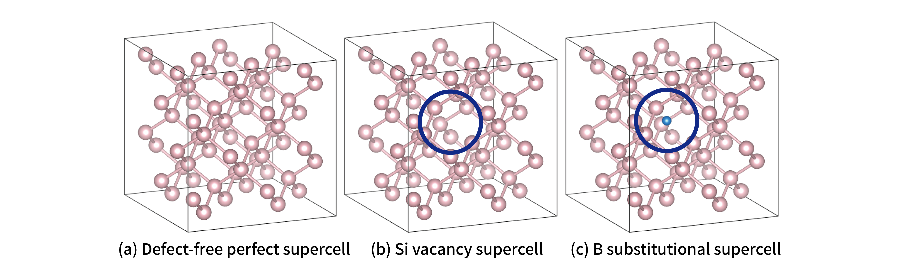### 2. Si-SiO2 Interface Model

Fig. 4 shows the atomistic slab structure of Si-SiO2 interface models. In the previous simulation studies, α-quartz or β-cristobalite structures were used for interface simulations[25,26]. In this study, we use α-quartz (1000)/silicon(100) slab model (see Fig. 4(a)). The optimized lattice constants of atomistic models are obtained from the materials projects database. Native vacancy defects are assumed to be the major interface defects which are formed at the semiconductoroxide interface, so the Si and oxygen ($O$) vacancy ($V_{Si}$, $V_{o}$) defect models are used for the simulation. Since the surrouding enviroment of the defect may affect its electrical properties considerably, the calculation of the interface defect is performed independently from the bulk model. The defect is formed near the Si-SiO2 interface. Fig. 4(b) shows the location of defects. The PAW cutoff energy of 400 eV and $3 \times 3 \times 3 k$-points are applied for electron structure calculations. The Perdew-Burke-Ernzerhof (PBE) potential is used for geometry optimization and electronic structure calculation. Structural minimization is performed until all the atomic forces are less than $0.01 eV Å$. To compensate for bandgap underestimation problems by using PBE functional, band gap and defect energy correction method is used.

Fig. 4. The Si-SiO2 interface structure (a) The minimum structure cells building the interface structure, (b) The position where the defect is formed on the interface structure. The defect is located at Si-SiO2 molecules bond.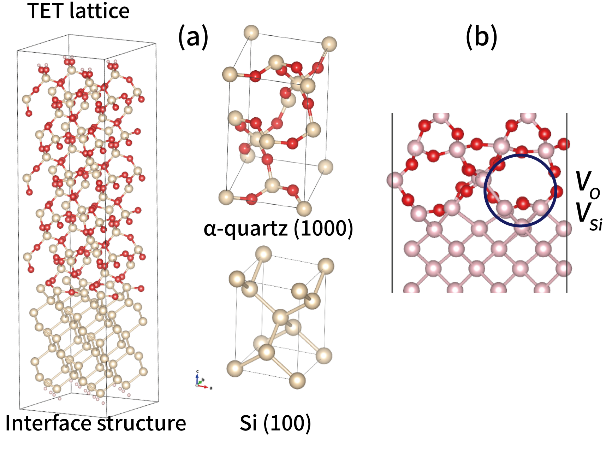## IV. CALCULATION RESULTS

### 1. Silicon Bulk Model

Fig. 5(a) shows the formation energies for a silicon vacancy ($V_{Si}$) and a boron substitutional ($Si : B$). The thick lines indicate the energetically minimal charge states for a given Fermi level. Fig. 5(b) shows the charged transition levels, which are intersections in Fig. 5(a). The ($+/ 0$)) transition level of the $V_{Si}$ structure and the ($0 /-$)) transition level of the $S i : B$ structure are close to the valence band maximum. The energy distances from the valence band maximum are $15 meV$ for the $V_{S i}$ structure and $117 meV$ for the $Si : B$ structure, respectively.

Fig. 5. (a) Calculated formation energy versus the Fermi energy level for Si vacancy ( $V_{Si}$ ) and substitutional B ( $Si : B$ ) in Si supercell, (b) Charged transition levels.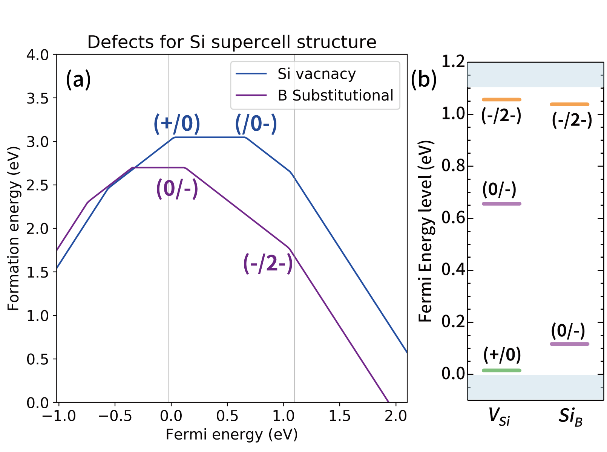In Fig. 6, the configuration coordinate (CC) diagram is plotted for the $Si : B$ system. The generalized coordinate $Q$ is the mass-weighted CC of the defect for the Cartesian coordinate system $R$.

Fig. 6. Calculated configuration coordinate (CC) diagram for the hole captured substitutional B ( $Si : B$ ) in the Si supercell system. Symbols are calculated values from the DFT simulation. Quadratic lines are fitted to the calculated values. Each curve represents the neutral ground state (black line) and the hole captured excited state (purple line). $\Delta E_{Si}$ is the energy difference between minima of two curves. It represents the same theoretical concept as minimal transition levels in bandgap (Fig. 2) and energy difference of two electronic states (Eq. (3)).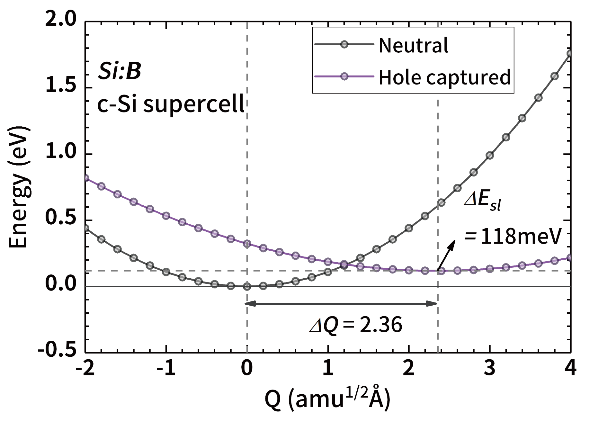The ground state (bottom black line and symbol) is the non-charged neutral state for the $Si: B$ supercell. The excited state (top purple line and symbol) is corresponding to the defect in the negatively charged state ($- / 0$) and a hole in the valence band. $\delta Q$ is the total sum of relaxations of neighbor atoms around the defect site. The energy difference between two minimum points of the curve is equivalent to the difference between the charged transition level and the valence band maximum in the formation energy diagram (see Fig. 5). $\Delta E_{s l}(Si : B)=118 meV$ from the supercell calculation, and the CC offset of two states is $\Delta Q=2.36 a m u^{\frac{1}{2}} Å$ . The quadratic fitting model (lines) comes from a reference paper.

Fig. 7 shows calculated capture cross sections and experimental values for the Si bulk supercell. The hole capture cross section is obtained by $\sigma_{p}=C_{p} / v_{p}$ ( $v_{p}$ is the mean thermal velocity of holes). When the temperature is increased, the MPE transition is increased because more energetic phonon branches are activated. Both of Fig. 7(a) and Fig. 7(b) show an inverse correlation between the decrease in temperature and the value of the hole capture cross sections ( $\sigma_{p}$ ). At T=300K, we get $\sigma_{p}$=2.26×10-18 cm2 for $V _{Si}$ and $\sigma_{p}$=2.01×10-18 cm2 for $Si : B$. The calculated cross section ( $\sigma_{p}$ ) is about one order-of-magnitude smaller than experimental results [31-33]. One possible cause of such discrepancy is the gamma point sampling employed in this work. Further investigation with additional sampling points would be required. It is also noted that similar results are also found in some calculation results in the existing reference of first principles based MPE calculations[8,17,18].

Fig. 7. Calculated non-radiative hole capture cross sections as functions of 1000/T (K-1) for (a) $V _{Si}$ , (b) $Si : B$ defects in Si supercell. The stars are experimental data taken from [31-33][31-33].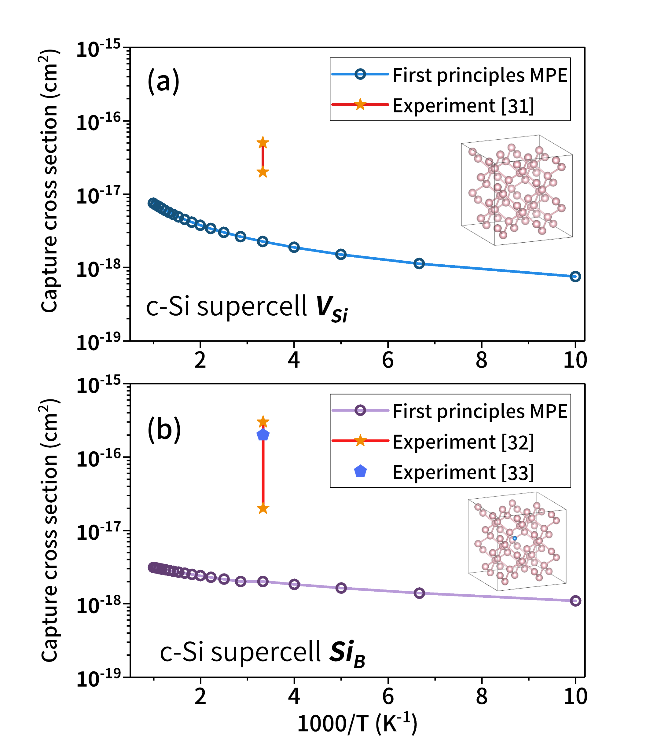### 2. Si-SiO2 Interface Model

Fig. 8 shows the calculated formation energies in the Si-SiO2 interface structure. Since the charged transition levels of Si vacancy are below the valence band maximum, we cannot use these transition levels for our calculations. Instead, in the case of the Si-SiO2 model, the capture parameter is obtained as a function of charged transition level ($\Delta E$).

Fig. 8. Calculated formation energy versus Fermi energy level for Si and O vacancies ($V_{o}$, $V_{Si}$) in the Si-SiO2 interface structure.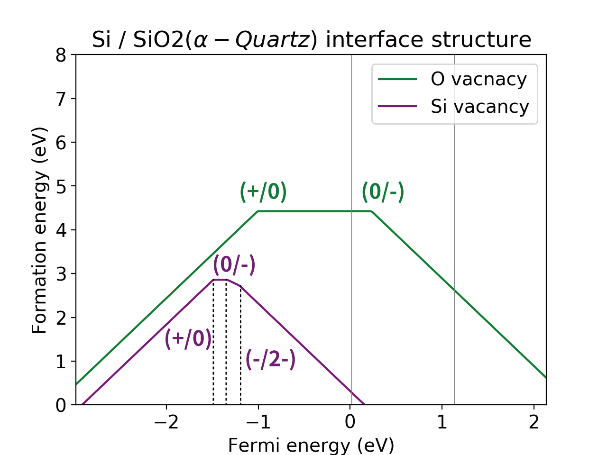Fig. 9 shows the hole capture cross section of each defect type according to the value of the charged transition level ($\Delta E$). As $\Delta E$ increases, the hole capture cross sections are decreased logarithmically. As the $\Delta E$ value increases, the phonon energy required for the MPE increases, so the capture cross section decreases. The total MPE is defined as a sum of hole capture cross sections of two defect models, $V_{o}$ and $V_{Si}$. The calculated capture cross section values are smaller than the experimental values[34,35]. This tendency is the same as the result of the Si bulk supercell cases.

Fig. 9. Calculated non-radiative hole capture cross section of O and Si vacancy ($V_{o}$, $V_{Si}$) defects in Si-SiO2 interface structure.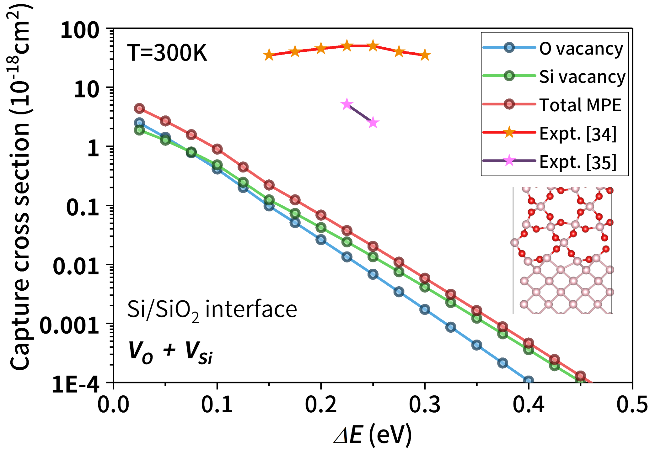## V. CONCLUSION

We have performed the first principles-based non-radiative MPE calculations for the Si bulk and the Si-SiO2 interface supercell structures. Using the method based on the adiabatic approximation, the hole capture cross section is calculated in those structures. Comparison with the experimental results reveals that the calculated capture cross section shows physically sound behaviors with significantly smaller magnitude. Considering more k-points sampling and phonon modes, better agreement with the experimental results is expected.

It is stressed that our calculation does not depend on the parameters obtained from the experiments for the trap characterization. The authors hope that this approach can be further developed to analyze the physical effects of defects in semiconductor materials and semiconductor device interfaces.

### ACKNOWLEDGMENTS

This work was supported by the Basic Science Research Program (Grant No. 2016R1C1B1014852) through the National Research Foundation of Korea funded by the Ministry of Science, ICT, and Future Planning.

### REFERENCES

1
Fleetwood D. M., Schrimpf R. D., 2008, Defects in microelectronic materials and devices, CRC press2
Mahapatra S., et al , 2013, A comparative study of different physics-based NBTI models, IEEE Transactions on Electron Devices, Vol. 60, No. 3, pp. 901-9163
Franco J., et al , 2012, Impact of single charged gate oxide defects on the performance and scaling of nanoscaled FETs, Reliability Physics Symposium (IRPS)4
Abakumov V. N., Perel V. I., Yassievich I. N., 1991, Nonradiative recombination in semiconductors, Elsevier, Vol. 335
Tsuchiya T., 2011, Interactions between interface traps in electron capture/emission processes: deviation from charge pumping current based on the Shockley–Read–Hall theory, Applied Physics Express, Vol. 4, No. 9, pp. 0941046
Huang K., Rhys A., 1950, Theory of light absorption and non-radiative transitions in F-centres, Proc. R. Soc. Lond. A, Vol. 204, No. 1078, pp. 406-4237
Henry C. H., Vo Lang D., 1977, Nonradiative capture and recombination by multiphonon emission in GaAs and GaP, Physical Review B, Vol. 15, pp. 9898
Shi L., Xu K., Wang L. W., 2015, Comparative study of ab initio nonradiative recombination rate calculations under different formalisms, Physical Review B, Vol. 91, pp. 2053159
Pässler R., 1989, Comparison between static and adiabatic coupling mechanisms for nonradiative multiphonon transitions in semiclassical approximation I. Tunnelling at small relaxation, Czechoslovak Journal of Physics B, Vol. 39, No. 2, pp. 155-19510
Giustino F., 2017, Electron-phonon interactions from first principles, Reviews of Modern Physics, Vol. 89, pp. 01500311
Freysoldt C., et al , 2014, First-principles calculations for point defects in solids, Reviews of modern physics, Vol. 86, pp. 25312
Lyons J. L., Janotti A., Van de Walle C. G., 2014, Effects of carbon on the electrical and optical properties of InN, GaN, and AlN, Physical Review B, Vol. 89, pp. 03520413
Dreyer C. E., et al , 2016, Gallium vacancy complexes as a cause of Shockley-Read-Hall recombination in III-nitride light emitters, Applied Physics Letters, Vol. 108, No. 14, pp. 14110114
Lyons J. L., Van de Walle Chris G., 2017, Computationally predicted energies and properties of defects in GaN, NPJ Computational Materials, Vol. 3, No. 1, pp. 1215
Giustino F., 2014, Materials modelling using density functional theory: properties and predictions, Oxford University Press16
Burke K., 2012, Perspective on density functional theory, The Journal of chemical physics, Vol. 136, No. 15, pp. 15090117
Shi L., Wang L. W., 2012, Ab initio calculations of deep-level carrier nonradiative recombination rates in bulk semiconductors, Physical review letters, Vol. 109, No. 24, pp. 24550118
Alkauskas A., Yan Q., Van de Walle C. G., 2014, First-principles theory of nonradiative carrier capture via multiphonon emission, Physical Review B, Vol. 90, pp. 07520219
Kresse G., Furthmüller J., 1996, Efficient iterative schemes for ab initio total-energy calculations using a plane-wave basis set, Physical review B, Vol. 54, pp. 1116920
Togo A., Tanaka I., 2015, First principles phonon calculations in materials science, Scripta Materialia, Vol. 108, pp. 1-521
Ong S. P., et al , 2013, Python Materials Genomics (pymatgen): A robust, open-source python library for materials analysis, Computational Materials Science, Vol. 68, pp. 314-31922
Broberg D., et al , 2016, PyCDT: A Python toolkit for modeling point defects in semiconductors and insulators, arXiv:1611.0748123
Freysoldt C., et al , 2009, Fully ab initio finite-size corrections for charged-defect supercell calculations, Physical review letters, Vol. 102, pp. 01640224
Heyd J., et al , 2003, Hybrid functionals based on a screened Coulomb potential, The Journal of chemical physics, Vol. 118, No. 18, pp. 8207-821525
Tsetseris L., Pantelides S. T., 2006, Oxygen Migration, Agglomeration, and Trapping: Key Factors for the Morphology of the Si−SiO2 Interface, Physical review letters, Vol. 97, pp. 11610126
Ribeiro Jr M., RC Fonseca L., Ferreira L. G., 2009, Accurate prediction of the Si/SiO2 interface band offset using the self-consistent ab initio DFT/LDA-1/2 method, Physical Review B, Vol. 79, pp. 24131227
Jain Anubhav, et al , 2013, Commentary: The Materials Project: A materials genome approach to accelerating materials innovation, Apl Materials, Vol. 1, No. 1, pp. 01100228
Perdew J. P., Burke K., Ernzerhof M., 1996, Generalized gradient approximation made simple, Physical review letters, Vol. 77, pp. 386529
Alkauskas A., Broqvist P., Pasquarello A., 2008, Defect energy levels in density functional calculations: Alignment and band gap problem, Physical review letters, Vol. 101, pp. 04640530
Schanovsky F., Goes W., Grasser T., 2011, Multiphonon hole trapping from first principles, J. Vac. Sci. Technol.: Materials, Processing, Measurement, and Phenomena, Vol. 29, No. 1, pp. 01A20131
Madelung O., Rössler U., Schulz M., 2002, Impurities and Defects in Group IV Elements, IV-IV and III-V Compounds. Part a: Group IV Elements, Numerical Data and Functional Relationships in Science and Technology–New Series, Group III Condensed Matter, Vol. 41, pp. 87732
Mooney P. M., et al , 1977, Defect energy levels in boron-doped silicon irradiated with 1-MeV electrons, Physical Review B, Vol. 15, pp. 383633
Troxell J. R., Watkins G. D., 1980, Interstitial boron in silicon: A negative-U system, Physical Review B, Vol. 22, pp. 92134
Kirton M. J., Uren M., 1986, Capture and emission kinetics of individual Si: SiO2 interface states, Applied physics letters, Vol. 48, No. 19, pp. 1270-127235
Saks N. S., Ancona M. G., 1990, Determination of interface trap capture cross sections using three-level charge pumping, IEEE Electron Device Letters, Vol. 11, No. 8, pp. 339-341## Author

received the B.S. degree in electrical engineering and the Ph.D. degree in electrical engineering and computer science from Seoul National University, Seoul, Korea, in 2001 and 2007, respectively.

He is currently an Assistant Professor with the School of Electrical Engineering and Computer Science, Gwangju Institute of Science and Technology, Gwangju, Korea.

His main research interests include physics-based device modeling.

received the B.Eng. degree in electrical engineering from Kyungpook National University, Daegu, Korea, in 2015 and is currently working toward the Ph.D. degree in electrical engineering from Gwangju Institute of Science and Technology (GIST), Gwangju, Korea.

His main research interests are defect-related physical phenomena in MOS interface of modern semiconductor devices.